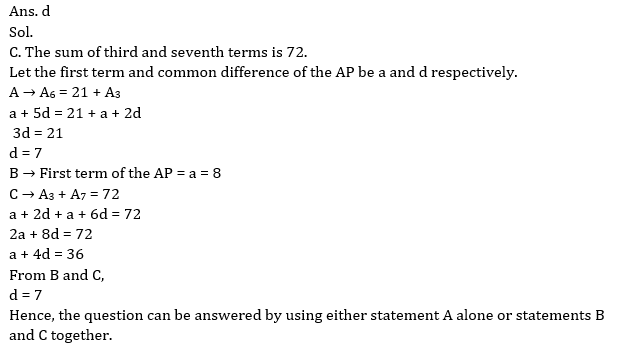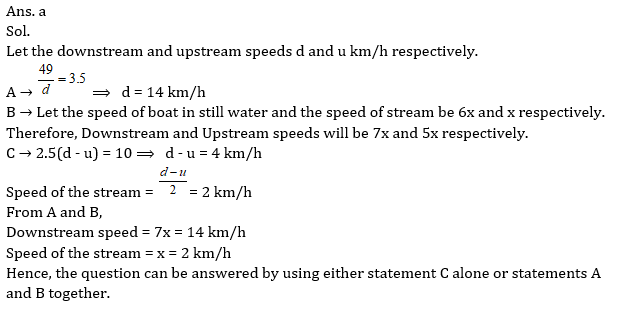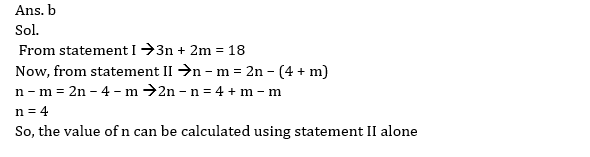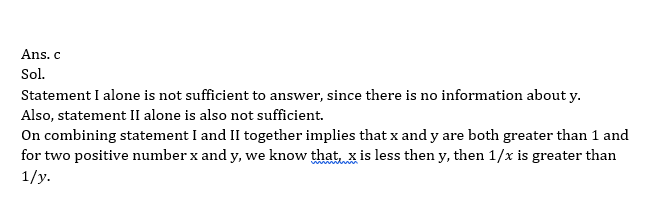# Data sufficiency Quiz for RRB PO & RRB CLERK : Quiz – 5

## Data sufficiency Quiz for RRB PO & RRB CLERK : Quiz – 5

Data Sufficiency plays a significant role in Quantitative Aptitude Section of banking exams such as IBPS, SBI and RBI PO and Clerk. You will get at least 4-5 questions from Data Sufficiency in one of IBPS, SBI and RBI PO & clerk exam. So, aspirants should focus on Data Sufficiency questions in detail. Here, we are providing you with the Data Sufficiency questions quiz with the detailed solution so that you can easily prepare for Data Sufficiency questions. We are providing here all-important latest pattern-based questions and Previous Year Questions of Data Sufficiency of various Government Exam like IBPS, SBI, and RBI PO and Clerk exam. This Data Sufficiency quiz we are providing is free. Attempt this Data Sufficiency quiz to practice important questions with answers and solutions. And score better in IBPS, SBI and RBI PO and Clerk exam.

Data Sufficiency Quiz to improve your Quantitative Aptitude for SBI Po & SBI clerk exam, IBPS PO & IBPS Clerk exam, IBPS RRB PO and assistant exam, LIC AAO, LIC Assistant and other competitive exam.

Directions (1 – 10): Each question below is followed by two or three statements I or A, II or B, and III or C. You have to determine whether the data given in the statements are sufficient for answering the question. You should use the data and your knowledge of Mathematics to choose between the possible answers. Read the statements and Answer.
1. What is the common difference of an arithmetic progression?
A. Sixth term of the AP is 21 more than the third term of the AP.
B. The first term of the AP is 8.
C. The sum of third and seventh terms is 72.
(a) Only A alone
(b) Only C alone
(c) Only B and C together
(d) Either A alone or B and C together
(e) Only B alone2. What is the speed of the stream?
A. The boat goes 49 km downstream in 3.5 hours.
B. The ratio of the speed of boat in still water and the speed of stream is 6:1.
C. The boat goes 10 km more in downstream than in upstream in 2.5 hours.
(a) Either C alone or A and B together
(b) Only A and B together
(c) Only A alone
(d) Only B alone
(e) Only C alone3. What is the value of a two-digit number?
A. The ratio of the value of the number and the sum of the digits of that number is 4 : 1.
B. The sum of the original number and the number obtained after interchanging the digits is 66.
C. The number obtained after interchanging the digits is 18 more than the number itself.
(a) Only A and B together
(b) Only B and C together
(c) Only A and C together
(d) All the three together
(e) Any two of the three together4. Find out the length of a platform.
A. A Train moving with a speed of 72 km/h crosses the platform in 50 sec.
B. Ratio of the lengths of the train and the platform is 2 : 3.
C. The length of the train is 400 meters.
(a) Any two of the three together
(b) All the three together are not sufficient
(c) Only A and B together
(d) Only B and C together
(e) Only A and C together5. What is the ratio of the efficiency of a man and a women?
A. 10 men are as efficient as 12 women.
B. 15 women can complete half of the work done by 25 men in same time.
C. A man is 20% more efficient than a woman.
(a) Only A alone
(b) Either A alone or C alone
(c) Any one of the three alone
(d) Only C alone
(e) Either A alone or B alone6. What is the value of n?
I. 3n + 2m = 18
II. n – m = 2n – (4+m)
(a) Only I
(b) Only II
(c) Both I and II
(d) Either I or II
(e) Cannot be determined7. Find out the length of train A.
I. Train A crosses another train B moving in the opposite direction in 10 sec.
II. Ratio of the speeds of trains A and B is 1 : 2.
II. Length of train B is 25% more than that of train A.
(a) All the three together are not sufficient
(b) Only I and II together
(c) All the three together
(d) Only I and II together
(e) Only II and III together8. What is the present age of Sabir?
I. The present age of Sabir is half of his father’s age.
II. After five years, the ratio of ages of Sabir and his father is 6 : 11.
III. Sabir is younger to his brother by five years.
(a) I and II
(b) I and III
(c) II and III
(d) All of these
(e) Cannot be determined9. Is 1/x greater than 1/y?
I. x is greater than 1.
II. x is less than y.
(a) Only I
(b) Only II
(c) Both I and II
(d) Either I or II
(e) Cannot be determined10. What will be the share of Q in the profit earned by P, Q and R together?
I. P, Q and R invested total amount of Rs. 25000 for a period of two years.
II. The profit earned at the end of two years is 30%.
III. The amount invested by Q is equal to the amount invested by P and R together.
(a) Only I
(b) Only II
(c) Only III
(d) All I, II and III are required to answer the question.
(e) None of these#### Attempt Quantitative Aptitude Topic Wise Online Test Series

Recommended PDF’s for :

#### Most important PDF’s for Bank, SSC, Railway and Other Government Exam : Download PDF Now

AATMA-NIRBHAR Series- Static GK/Awareness Practice Ebook PDF Get PDF here
The Banking Awareness 500 MCQs E-book| Bilingual (Hindi + English) Get PDF here
AATMA-NIRBHAR Series- Banking Awareness Practice Ebook PDF Get PDF here
Computer Awareness Capsule 2.O Get PDF here
AATMA-NIRBHAR Series Quantitative Aptitude Topic-Wise PDF Get PDF here
AATMA-NIRBHAR Series Reasoning Topic-Wise PDF Get PDF Here
Memory Based Puzzle E-book | 2016-19 Exams Covered Get PDF here
Caselet Data Interpretation 200 Questions Get PDF here
Puzzle & Seating Arrangement E-Book for BANK PO MAINS (Vol-1) Get PDF here
ARITHMETIC DATA INTERPRETATION 2.O E-book Get PDF here

3